Chemistry: Atoms First 2e

# Exercises

### 6.1Formula Mass

1.

What is the total mass (amu) of carbon in each of the following molecules?

(a) CH4

(b) CHCl3

(c) C12H10O6

(d) CH3CH2CH2CH2CH3

2.

What is the total mass of hydrogen in each of the molecules?

(a) CH4

(b) CHCl3

(c) C12H10O6

(d) CH3CH2CH2CH2CH3

3.

Calculate the molecular or formula mass of each of the following:

(a) P4

(b) H2O

(c) Ca(NO3)2

(d) CH3CO2H (acetic acid)

(e) C12H22O11 (sucrose, cane sugar)

4.

Determine the molecular mass of the following compounds:

(a)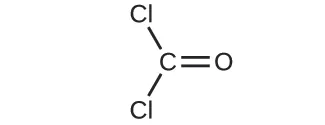(b)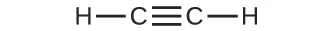(c)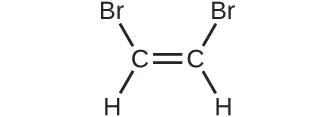(d)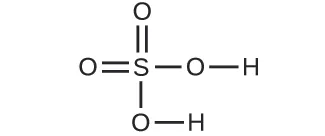5.

Determine the molecular mass of the following compounds:

(a)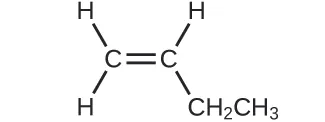(b)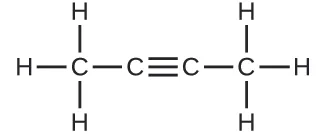(c)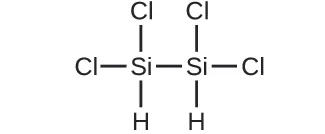(d)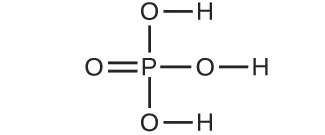6.

Which molecule has a molecular mass of 28.05 amu?

(a)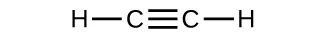(b)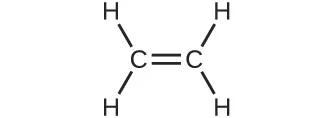(c)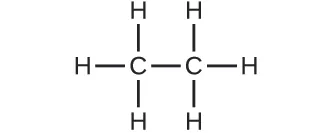### 6.2Determining Empirical and Molecular Formulas

7.

What information is needed to determine the molecular formula of a compound from the empirical formula?

8.

Calculate the following to four significant figures:

(a) the percent composition of ammonia, NH3

(b) the percent composition of photographic fixer solution “hypo,” Na2S2O3

(c) the percent of calcium ion in Ca3(PO4)2

9.

Determine the following to four significant figures:

(a) the percent composition of hydrazoic acid, HN3

(b) the percent composition of TNT, C6H2(CH3)(NO2)3

(c) the percent of SO42– in Al2(SO4)3

10.

Determine the percent ammonia, NH3, in Co(NH3)6Cl3, to three significant figures.

11.

Determine the percent water in CuSO4∙5H2O to three significant figures.

12.

Determine the empirical formulas for compounds with the following percent compositions:

(a) 15.8% carbon and 84.2% sulfur

(b) 40.0% carbon, 6.7% hydrogen, and 53.3% oxygen

13.

Determine the empirical formulas for compounds with the following percent compositions:

(a) 43.6% phosphorus and 56.4% oxygen

(b) 28.7% K, 1.5% H, 22.8% P, and 47.0% O

14.

A compound of carbon and hydrogen contains 92.3% C and has a molar mass of 78.1 g/mol. What is its molecular formula?

15.

Dichloroethane, a compound that is often used for dry cleaning, contains carbon, hydrogen, and chlorine. It has a molar mass of 99 g/mol. Analysis of a sample shows that it contains 24.3% carbon and 4.1% hydrogen. What is its molecular formula?

16.

Determine the empirical and molecular formula for chrysotile asbestos. Chrysotile has the following percent composition: 28.03% Mg, 21.60% Si, 1.16% H, and 49.21% O. The molar mass for chrysotile is 520.8 g/mol.

17.

Polymers are large molecules composed of simple units repeated many times. Thus, they often have relatively simple empirical formulas. Calculate the empirical formulas of the following polymers:

(a) Lucite (Plexiglas); 59.9% C, 8.06% H, 32.0% O

(b) Saran; 24.8% C, 2.0% H, 73.1% Cl

(c) polyethylene; 86% C, 14% H

(d) polystyrene; 92.3% C, 7.7% H

(e) Orlon; 67.9% C, 5.70% H, 26.4% N

18.

A major textile dye manufacturer developed a new yellow dye. The dye has a percent composition of 75.95% C, 17.72% N, and 6.33% H by mass with a molar mass of about 240 g/mol. Determine the molecular formula of the dye.

### 6.3Molarity

19.

Explain what changes and what stays the same when 1.00 L of a solution of NaCl is diluted to 1.80 L.

20.

What information is needed to calculate the molarity of a sulfuric acid solution?

21.

A 200-mL sample and a 400-mL sample of a solution of salt have the same molarity. In what ways are the two samples identical? In what ways are these two samples different?

22.

Determine the molarity for each of the following solutions:

(a) 0.444 mol of CoCl2 in 0.654 L of solution

(b) 98.0 g of phosphoric acid, H3PO4, in 1.00 L of solution

(c) 0.2074 g of calcium hydroxide, Ca(OH)2, in 40.00 mL of solution

(d) 10.5 kg of Na2SO4·10H2O in 18.60 L of solution

(e) 7.0 $××$ 10−3 mol of I2 in 100.0 mL of solution

(f) 1.8 $××$ 104 mg of HCl in 0.075 L of solution

23.

Determine the molarity of each of the following solutions:

(a) 1.457 mol KCl in 1.500 L of solution

(b) 0.515 g of H2SO4 in 1.00 L of solution

(c) 20.54 g of Al(NO3)3 in 1575 mL of solution

(d) 2.76 kg of CuSO4·5H2O in 1.45 L of solution

(e) 0.005653 mol of Br2 in 10.00 mL of solution

(f) 0.000889 g of glycine, C2H5NO2, in 1.05 mL of solution

24.

Consider this question: What is the mass of the solute in 0.500 L of 0.30 M glucose, C6H12O6, used for intravenous injection?

(a) Outline the steps necessary to answer the question.

25.

Consider this question: What is the mass of solute in 200.0 L of a 1.556-M solution of KBr?

(a) Outline the steps necessary to answer the question.

26.

Calculate the number of moles and the mass of the solute in each of the following solutions:

(a) 2.00 L of 18.5 M H2SO4, concentrated sulfuric acid

(b) 100.0 mL of 3.8 $××$ 10−6 M NaCN, the minimum lethal concentration of sodium cyanide in blood serum

(c) 5.50 L of 13.3 M H2CO, the formaldehyde used to “fix” tissue samples

(d) 325 mL of 1.8 $××$ 10−6 M FeSO4, the minimum concentration of iron sulfate detectable by taste in drinking water

27.

Calculate the number of moles and the mass of the solute in each of the following solutions:

(a) 325 mL of 8.23 $××$ 10−5 M KI, a source of iodine in the diet

(b) 75.0 mL of 2.2 $××$ 10−5 M H2SO4, a sample of acid rain

(c) 0.2500 L of 0.1135 M K2CrO4, an analytical reagent used in iron assays

(d) 10.5 L of 3.716 M (NH4)2SO4, a liquid fertilizer

28.

Consider this question: What is the molarity of KMnO4 in a solution of 0.0908 g of KMnO4 in 0.500 L of solution?

(a) Outline the steps necessary to answer the question.

29.

Consider this question: What is the molarity of HCl if 35.23 mL of a solution of HCl contain 0.3366 g of HCl?

(a) Outline the steps necessary to answer the question.

30.

Calculate the molarity of each of the following solutions:

(a) 0.195 g of cholesterol, C27H46O, in 0.100 L of serum, the average concentration of cholesterol in human serum

(b) 4.25 g of NH3 in 0.500 L of solution, the concentration of NH3 in household ammonia

(c) 1.49 kg of isopropyl alcohol, C3H7OH, in 2.50 L of solution, the concentration of isopropyl alcohol in rubbing alcohol

(d) 0.029 g of I2 in 0.100 L of solution, the solubility of I2 in water at 20 °C

31.

Calculate the molarity of each of the following solutions:

(a) 293 g HCl in 666 mL of solution, a concentrated HCl solution

(b) 2.026 g FeCl3 in 0.1250 L of a solution used as an unknown in general chemistry laboratories

(c) 0.001 mg Cd2+ in 0.100 L, the maximum permissible concentration of cadmium in drinking water

(d) 0.0079 g C7H5SNO3 in one ounce (29.6 mL), the concentration of saccharin in a diet soft drink.

32.

There is about 1.0 g of calcium, as Ca2+, in 1.0 L of milk. What is the molarity of Ca2+ in milk?

33.

What volume of a 1.00-M Fe(NO3)3 solution can be diluted to prepare 1.00 L of a solution with a concentration of 0.250 M?

34.

If 0.1718 L of a 0.3556-M C3H7OH solution is diluted to a concentration of 0.1222 M, what is the volume of the resulting solution?

35.

If 4.12 L of a 0.850 M-H3PO4 solution is be diluted to a volume of 10.00 L, what is the concentration of the resulting solution?

36.

What volume of a 0.33-M C12H22O11 solution can be diluted to prepare 25 mL of a solution with a concentration of 0.025 M?

37.

What is the concentration of the NaCl solution that results when 0.150 L of a 0.556-M solution is allowed to evaporate until the volume is reduced to 0.105 L?

38.

What is the molarity of the diluted solution when each of the following solutions is diluted to the given final volume?

(a) 1.00 L of a 0.250-M solution of Fe(NO3)3 is diluted to a final volume of 2.00 L

(b) 0.5000 L of a 0.1222-M solution of C3H7OH is diluted to a final volume of 1.250 L

(c) 2.35 L of a 0.350-M solution of H3PO4 is diluted to a final volume of 4.00 L

(d) 22.50 mL of a 0.025-M solution of C12H22O11 is diluted to 100.0 mL

39.

What is the final concentration of the solution produced when 225.5 mL of a 0.09988-M solution of Na2CO3 is allowed to evaporate until the solution volume is reduced to 45.00 mL?

40.

A 2.00-L bottle of a solution of concentrated HCl was purchased for the general chemistry laboratory. The solution contained 868.8 g of HCl. What is the molarity of the solution?

41.

An experiment in a general chemistry laboratory calls for a 2.00-M solution of HCl. How many mL of 11.9 M HCl would be required to make 250 mL of 2.00 M HCl?

42.

What volume of a 0.20-M K2SO4 solution contains 57 g of K2SO4?

43.

The US Environmental Protection Agency (EPA) places limits on the quantities of toxic substances that may be discharged into the sewer system. Limits have been established for a variety of substances, including hexavalent chromium, which is limited to 0.50 mg/L. If an industry is discharging hexavalent chromium as potassium dichromate (K2Cr2O7), what is the maximum permissible molarity of that substance?

### 6.4Other Units for Solution Concentrations

44.

Consider this question: What mass of a concentrated solution of nitric acid (68.0% HNO3 by mass) is needed to prepare 400.0 g of a 10.0% solution of HNO3 by mass?

(a) Outline the steps necessary to answer the question.

45.

What mass of a 4.00% NaOH solution by mass contains 15.0 g of NaOH?

46.

What mass of solid NaOH (97.0% NaOH by mass) is required to prepare 1.00 L of a 10.0% solution of NaOH by mass? The density of the 10.0% solution is 1.109 g/mL.

47.

What mass of HCl is contained in 45.0 mL of an aqueous HCl solution that has a density of 1.19 g cm–3 and contains 37.21% HCl by mass?

48.

The hardness of water (hardness count) is usually expressed in parts per million (by mass) of CaCO3, which is equivalent to milligrams of CaCO3 per liter of water. What is the molar concentration of Ca2+ ions in a water sample with a hardness count of 175 mg CaCO3/L?

49.

The level of mercury in a stream was suspected to be above the minimum considered safe (1 part per billion by weight). An analysis indicated that the concentration was 0.68 parts per billion. Assume a density of 1.0 g/mL and calculate the molarity of mercury in the stream.

50.

In Canada and the United Kingdom, devices that measure blood glucose levels provide a reading in millimoles per liter. If a measurement of 5.3 mM is observed, what is the concentration of glucose (C6H12O6) in mg/dL?

51.

A throat spray is 1.40% by mass phenol, C6H5OH, in water. If the solution has a density of 0.9956 g/mL, calculate the molarity of the solution.

52.

Copper(I) iodide (CuI) is often added to table salt as a dietary source of iodine. How many moles of CuI are contained in 1.00 lb (454 g) of table salt containing 0.0100% CuI by mass?

53.

A cough syrup contains 5.0% ethyl alcohol, C2H5OH, by mass. If the density of the solution is 0.9928 g/mL, determine the molarity of the alcohol in the cough syrup.

54.

D5W is a solution used as an intravenous fluid. It is a 5.0% by mass solution of dextrose (C6H12O6) in water. If the density of D5W is 1.029 g/mL, calculate the molarity of dextrose in the solution.

55.

Find the molarity of a 40.0% by mass aqueous solution of sulfuric acid, H2SO4, for which the density is 1.3057 g/mL.

Order a print copy

As an Amazon Associate we earn from qualifying purchases.